# DeepLearning-Lec8-Notes

(Tim Lee) #1

Hi All,

I’m looking forward to the topics in part 2 of deep learning. While I think Jeremy’s notebooks are pretty self-explanatory this week, I’ve uploaded my notes into the forum as well. Hope its helpful!

• Tim

## Welcome to Lesson 8

### Where we are:

1. Differentiable Layers - coined by Yann Lecun, we don’t call it deep learning but differentiable programming. In part 1, we setup a diff’able function and a loss function that explains how good the parameters are. If you can define a loss function to score a task, and you have a flexible NN, you are done.

`````` Yeah, Differentiable Programming is little more than a rebranding of the modern collection Deep Learning techniques, the same way Deep Learning was a rebranding of the modern incarnations of neural nets with more than two layers.
The important point is that people are now building a new kind of software by assembling networks of parameterized functional blocks and by training them from examples using some form of gradient-based optimization….It’s really very much like a regular program, except it’s parameterized, automatically differentiated, and trainable/optimizable.
Yann LeCun, Director of FAIR
``````
2. Transfer Learning - you should never need to start on random data. You should only start if no one has every solved a problem that is remotely close or related to what you are trying to solve. Fastai’s focus is completely focused on transfer learning and as a result the library is much different than any other library. In short, transfer learning takes a trained model, takes away the last few layers, retrains the last few layers, fine tunes the entire architecture, and as a result, trains a lot faster and requires less data!

3.Architecture Design - There’s only a few models that generally cover a majority of problems. In part 1, we generally focused on activation functions and the output. We spend less time talking about architecture.

1. how to Avoid overfitting - Create something that is over-parameterized. Train it for sure, overfit it, which guarantees that there is a predictive capability. Then we use the following techniques to reduce the overfitting to arrive at a generalized model.
• Get more data
• Data augmentation
• Generalized Architecture
• regularization
• Lastly, reduce architecture complexity

5.Embeddings - Just earlier last year, there was fewer discussions of using embeddings in tabular data (vs. the NLP related topics) Now these days there are more and more examples of using embeddings in traditional structured tabular data settings

# Part 2’s Goals / Approach

Part 1 was an introduction to best practices. Overviewed mature techniques that were reasonably reliable for a wide variety of real world problems. These techniques were developed and tested over a longer period of time. Cutting Edge means that the best parameters may not always be evident. It may or may not be the absolute best solution, and the fastai implementation may still be buggy. The techniques covered will be promising in the research world, but may require tweaking. It’s exciting to work with the most current techniques and to also learn these recent techniques and understand what is going on vs. the recipe and pre-built libraries of pytorch and fastai.

#### Some caveats:

• Requires fastai customization
• Need to understand python well
• Other code resources will generally be research quality
• Code samples online nearly have always have problems
• Each lesson will be incomplete, ideas to explore

If you are considering building your own box checkout the forums.

# It’s time to start reading papers

Each week we will be implementing a paper. In academic papers love using greek letters. Academics never refactor or substitute, but equations can get very long. Academic papers can be weird, but its the current way that research is commuted these days.

#### Since this is all cutting-edge, its a great opportunity!

• make a blog post, explain things in plain language
• maybe a simple implementation that other people can use
• use a published case study and translate the technique to a similar problem

## Part 2’s Topics

#### Generative models

NN’s generally output numbers. But now the outputs will be locations of objects, or a complete picture with a class in every pixel. Or an enhanced super-resolution of an image. Or text translated into french. Requires some different ways of thinking about things and different architectures.

## Object Detection

### Introduction

#### 1. We are classifying Multiple things

This part is not new, as we have done this in part1, the planet tutorial.

#### 2. Finding Bounding boxes around the objects we are classifying

The box has the object entirely in it, but is no bigger than it needs to be. For these object detection datasets, we are looking for a pool of objects, but not necessarily EVERY object in the photo (mountain, tree cloud).

## Stages:

• 1 Find largest item
• 2 Find where it is
• 3 do both at the same time

## Stage 1: Find the largest object in the image

https://github.com/fastai/fastai/blob/master/courses/dl2/pascal.ipynb

``````%matplotlib inline
``````

#### Note, if you have a single GPU change the device to `.set_device(0)`

``````import sys
sys.path.append('../')
from fastai.conv_learner import *
from fastai.dataset import *

from pathlib import Path
import json
from PIL import ImageDraw, ImageFont
from matplotlib import patches, patheffects

# check to make sure you set the device
torch.cuda.set_device(0)
``````

## Pascal VOC

We will be looking at the Pascal VOC dataset. It’s quite slow, so you may prefer to download from this mirror. There are two different competition/research datasets, from 2007 and 2012. We’ll be using the 2007 version. You can use the larger 2012 for better results, or even combine them (but be careful to avoid data leakage between the validation sets if you do this).

Unlike previous lessons, we are using the python 3 standard library `pathlib` for our paths and file access. `pathlib` is the python3 library for interacting with files.

``````The pathlib module was first included in python 3.4 and has been enhanced in each of the subsequent releases. Pathlib is an object oriented interface to the filesystem and provides a more intuitive method to interact with the filesystem in a platform agnostic and pythonic manner.
``````

Cheat Sheet

Note that it returns an OS-specific class (on Linux, `PosixPath`) so your output may look a little different. Most libraries than take paths as input can take a pathlib object - although some (like `cv2`) can’t, in which case you can use `str()` to convert it to a string.

2. make a `data` dir
3. make a `pascal` dir
4. move the `.json` files out of the PASCAL_VOC into the `pascal/` dir
``````!wget http://pjreddie.com/media/files/VOCtrainval_06-Nov-2007.tar
``````
``````--2018-03-21 01:00:51--  http://pjreddie.com/media/files/VOCtrainval_06-Nov-2007.tar
Resolving pjreddie.com (pjreddie.com)... 128.208.3.39
Connecting to pjreddie.com (pjreddie.com)|128.208.3.39|:80... connected.
HTTP request sent, awaiting response... 301 Moved Permanently
Location: https://pjreddie.com/media/files/VOCtrainval_06-Nov-2007.tar [following]
--2018-03-21 01:00:51--  https://pjreddie.com/media/files/VOCtrainval_06-Nov-2007.tar
Connecting to pjreddie.com (pjreddie.com)|128.208.3.39|:443... connected.
HTTP request sent, awaiting response... 200 OK
Length: 460032000 (439M) [application/octet-stream]
Saving to: ‘VOCtrainval_06-Nov-2007.tar’

VOCtrainval_06-Nov- 100%[===================>] 438.72M  12.7MB/s    in 28s

2018-03-21 01:01:20 (15.5 MB/s) - ‘VOCtrainval_06-Nov-2007.tar’ saved [460032000/460032000]
``````
``````!wget https://storage.googleapis.com/coco-dataset/external/PASCAL_VOC.zip
``````
``````--2018-03-21 01:05:31--  https://storage.googleapis.com/coco-dataset/external/PASCAL_VOC.zip
HTTP request sent, awaiting response... 200 OK
Length: 1998182 (1.9M) [application/zip]
Saving to: ‘PASCAL_VOC.zip’

PASCAL_VOC.zip      100%[===================>]   1.91M  --.-KB/s    in 0.02s

2018-03-21 01:05:31 (77.5 MB/s) - ‘PASCAL_VOC.zip’ saved [1998182/1998182]
``````

#### We will be using Python3’s path lib, creating a generator around the directory

``````PATH = Path('data/pascal')
list(PATH.iterdir())
``````
``````[PosixPath('data/pascal/VOCdevkit'),
PosixPath('data/pascal/pascal_train2007.json'),
PosixPath('data/pascal/pascal_test2007.json'),
PosixPath('data/pascal/pascal_val2012.json'),
PosixPath('data/pascal/VOCtrainval_06-Nov-2007.tar'),
PosixPath('data/pascal/pascal_val2007.json'),
PosixPath('data/pascal/PASCAL_VOC.zip'),
PosixPath('data/pascal/pascal_train2012.json'),
PosixPath('data/pascal/PASCAL_VOC'),
PosixPath('data/pascal/models'),
PosixPath('data/pascal/src'),
PosixPath('data/pascal/tmp')]
``````

``````trn_j = json.load((PATH/'pascal_train2007.json').open())
trn_j.keys()
``````
``````dict_keys(['images', 'type', 'annotations', 'categories'])
``````

#### Image information

• `filename` - the related image with filename
• `height` - how big the height of the image is
• `width` - how big the width of the image is
• `id` - the image id for joining to other datasets
``````IMAGES,ANNOTATIONS,CATEGORIES = ['images', 'annotations', 'categories']
trn_j[IMAGES][:5]
``````
``````[{'file_name': '000012.jpg', 'height': 333, 'id': 12, 'width': 500},
{'file_name': '000017.jpg', 'height': 364, 'id': 17, 'width': 480},
{'file_name': '000023.jpg', 'height': 500, 'id': 23, 'width': 334},
{'file_name': '000026.jpg', 'height': 333, 'id': 26, 'width': 500},
{'file_name': '000032.jpg', 'height': 281, 'id': 32, 'width': 500}]
``````

#### Bounding Boxes

• `bbox`: column, row (top left) , height, width
• `id`: which image
• `category_id` : which label
• `segmentation` : ignore of this tutorail (polygon bounding
``````trn_j[ANNOTATIONS][:2]
``````
``````[{'area': 34104,
'bbox': [155, 96, 196, 174],
'category_id': 7,
'id': 1,
'ignore': 0,
'image_id': 12,
'iscrowd': 0,
'segmentation': [[155, 96, 155, 270, 351, 270, 351, 96]]},
{'area': 13110,
'bbox': [184, 61, 95, 138],
'category_id': 15,
'id': 2,
'ignore': 0,
'image_id': 17,
'iscrowd': 0,
'segmentation': [[184, 61, 184, 199, 279, 199, 279, 61]]}]
``````

#### Make a lookup from id to name

``````trn_j[CATEGORIES][:4]
``````
``````[{'id': 1, 'name': 'aeroplane', 'supercategory': 'none'},
{'id': 2, 'name': 'bicycle', 'supercategory': 'none'},
{'id': 3, 'name': 'bird', 'supercategory': 'none'},
{'id': 4, 'name': 'boat', 'supercategory': 'none'}]
``````

It’s helpful to use constants instead of strings, since we get tab-completion and don’t mistype.

``````FILE_NAME,ID,IMG_ID,CAT_ID,BBOX = 'file_name','id','image_id','category_id','bbox'

cats = dict((o[ID], o['name']) for o in trn_j[CATEGORIES])
trn_fns = dict((o[ID], o[FILE_NAME]) for o in trn_j[IMAGES])
trn_ids = [o[ID] for o in trn_j[IMAGES]]
``````

### Lets take a look at whats in the VOC 2007 dataset

``````list((PATH/'VOCdevkit'/'VOC2007').iterdir())
``````
``````[PosixPath('data/pascal/VOCdevkit/VOC2007/ImageSets'),
PosixPath('data/pascal/VOCdevkit/VOC2007/SegmentationObject'),
PosixPath('data/pascal/VOCdevkit/VOC2007/SegmentationClass'),
PosixPath('data/pascal/VOCdevkit/VOC2007/Annotations'),
PosixPath('data/pascal/VOCdevkit/VOC2007/JPEGImages')]
``````

#### Store the Image path

``````JPEGS = 'VOCdevkit/VOC2007/JPEGImages'
``````

#### Make a compound path

``````IMG_PATH = PATH/JPEGS
list(IMG_PATH.iterdir())[:5]
``````
``````[PosixPath('data/pascal/VOCdevkit/VOC2007/JPEGImages/006948.jpg'),
PosixPath('data/pascal/VOCdevkit/VOC2007/JPEGImages/005796.jpg'),
PosixPath('data/pascal/VOCdevkit/VOC2007/JPEGImages/007006.jpg'),
PosixPath('data/pascal/VOCdevkit/VOC2007/JPEGImages/004693.jpg'),
PosixPath('data/pascal/VOCdevkit/VOC2007/JPEGImages/002279.jpg')]
``````

Each image has a unique ID.

``````im0_d = trn_j[IMAGES]
im0_d[FILE_NAME],im0_d[ID]
``````
``````('000012.jpg', 12)
``````

A `defaultdict` is useful any time you want to have a default dictionary entry for new keys. Here we create a dict from image IDs to a list of annotations (tuple of bounding box and class id).

We convert VOC’s height/width into top-left/bottom-right, and switch x/y coords to be consistent with numpy.
We are swapping dimensions, to be consistent, numpy, ROWS x COLUMNS

Output

``````{
IMG_ID  : (array(top_left_row, top_left_col, lower_right_row, lower_right_col), CAT_ID)
}
``````
``````#initialize the default dictionary
trn_anno = collections.defaultdict(lambda:[])

# for each annotation
for o in trn_j[ANNOTATIONS]:

# if not ignore
if not o['ignore']:
# get the original bounding box information
bb = o[BBOX]
bb = np.array([bb, bb, bb+bb-1, bb+bb-1])

#
trn_anno[o[IMG_ID]].append((bb,o[CAT_ID]))

len(trn_anno)
``````
``````2501
``````

#### example 1

``````im0_a = im_a; im0_a
``````
``````(array([ 96, 155, 269, 350]), 7)
``````
``````cats
``````
``````'car'
``````

#### example 2

``````trn_anno
``````
``````[(array([ 61, 184, 198, 278]), 15), (array([ 77,  89, 335, 402]), 13)]
``````
``````cats,cats
``````
``````('person', 'horse')
``````

### Create a short function that will change a boundbox to height & width to translate data

``````def bb_hw(a): return np.array([a,a,a-a,a-a])
``````

We will use fast.ai’s `open_image` library to create an image to view

``````im = open_image(IMG_PATH/im0_d[FILE_NAME])
``````

#### Create a short function to show the image in the notebook

Matplotlib’s `plt.subplots` is a really useful wrapper for creating plots, regardless of whether you have more than one subplot. Note that Matplotlib has an optional object-oriented API which I think is much easier to understand and use (although few examples online use it!)

``````def show_img(im, figsize=None, ax=None):
if not ax: fig,ax = plt.subplots(figsize=figsize)
ax.imshow(im)
ax.get_xaxis().set_visible(False)
ax.get_yaxis().set_visible(False)
return ax
``````

#### Create an outlining function

A simple but rarely used trick to making text visible regardless of background is to use white text with black outline, or visa versa. Here’s how to do it in matplotlib.

``````def draw_outline(o, lw):
o.set_path_effects([patheffects.Stroke(
linewidth=lw, foreground='black'), patheffects.Normal()])
``````

Note that `*` in argument lists is the splat operator. In this case it’s a little shortcut compared to writing out `b[-2],b[-1]`.

#### Make a rectangle for the bounding box

``````def draw_rect(ax, b):
patch = ax.add_patch(patches.Rectangle(b[:2], *b[-2:], fill=False, edgecolor='white', lw=2))
draw_outline(patch, 4)
``````

#### Make a quick function to write the text label (for the category)

``````def draw_text(ax, xy, txt, sz=14):
text = ax.text(*xy, txt,
verticalalignment='top', color='white', fontsize=sz, weight='bold')
draw_outline(text, 1)
``````

### Let’s test out showing an image!

``````ax = show_img(im)
b = bb_hw(im0_a)
draw_rect(ax, b)
draw_text(ax, b[:2], cats[im0_a])
``````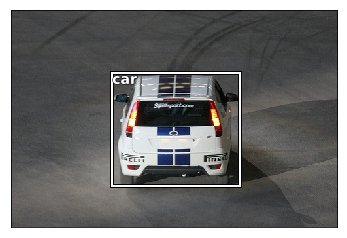### Let’s make a function to show multiple objects on a single image

``````def draw_im(im, ann):
ax = show_img(im, figsize=(16,8))
for b,c in ann:
b = bb_hw(b)
draw_rect(ax, b)
draw_text(ax, b[:2], cats[c], sz=16)
``````
``````def draw_idx(i):
im_a = trn_anno[i]
im = open_image(IMG_PATH/trn_fns[i])
print(im.shape)
draw_im(im, im_a)
``````
``````draw_idx(17)
``````
``````(364, 480, 3)
``````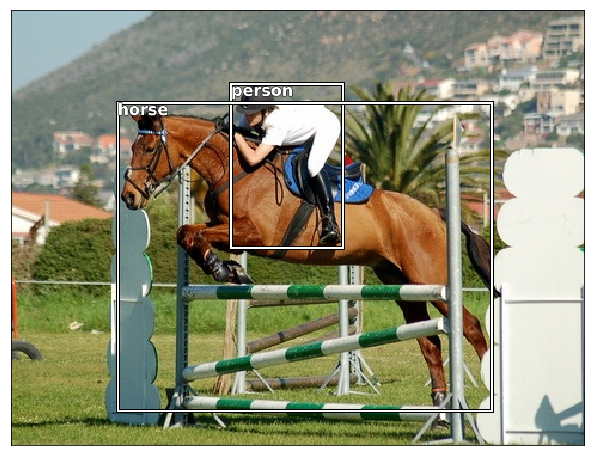# Next Complex step - Largest Item Classifier

A lambda function is simply a way to define an anonymous function inline. Here we use it to describe how to sort the annotation for each image - by bounding box size (descending).

This snippet sorts the objets

``````sorted(b, key=lambda x: np.product(x[-2:]-x[:2]), reverse=True)
``````

We subtract the upper left from the bottom right and multiply (`np.product`) the values to get an area.
lambda x: np.product(x[-2:]-x[:2])

``````def get_lrg(b):
if not b: raise Exception()
b = sorted(b, key=lambda x: np.product(x[-2:]-x[:2]), reverse=True)
return b
``````

### dictionary comprehension - storing the biggest objects:

``````{
IMG_ID : largest bounding box,
...
}
``````
``````trn_lrg_anno = {a: get_lrg(b) for a,b in trn_anno.items()}
``````
``````b,c = trn_lrg_anno
b = bb_hw(b)
ax = show_img(open_image(IMG_PATH/trn_fns), figsize=(5,10))
draw_rect(ax, b)
draw_text(ax, b[:2], cats[c], sz=16)
``````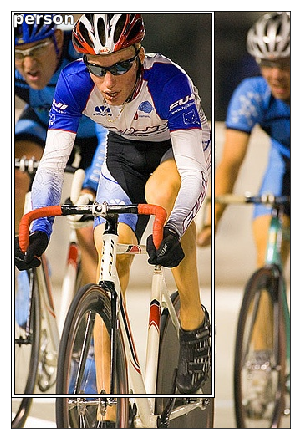### Let’s store the largest object per file in a `CSV` file

``````(PATH/'tmp').mkdir(exist_ok=True)
CSV = PATH/'tmp/lrg.csv'
``````

Often it’s easiest to simply create a CSV of the data you want to model, rather than trying to create a custom dataset. Here we use Pandas to help us create a CSV of the image filename and class.

``````df = pd.DataFrame({'fn': [trn_fns[o] for o in trn_ids],
'cat': [cats[trn_lrg_anno[o]] for o in trn_ids]}, columns=['fn','cat'])
df.to_csv(CSV, index=False)
``````

### Make a Resnet model to learn

#### Considerations

• The training images are different sized
• Since we are doing bounding boxes, many of the objects are near the edge, so we want to be careful not to cut the objects off the picture
• So we will put `CropType.NO` so we will “squish” instead
``````f_model = resnet34
sz=224
bs=64
``````

From here it’s just like Dogs vs Cats!

``````tfms = tfms_from_model(f_model, sz, aug_tfms=transforms_side_on, crop_type=CropType.NO)
md = ImageClassifierData.from_csv(PATH, JPEGS, CSV, tfms=tfms)
``````

#### A note on Data Loaders

A data loader is an iterator that will provide a mini-batch of data ( subset). But first we need to ensure that we start at the beginning of the dataset. Pythons’ `iter()` method will create an iterator object and start at the beginning of the dataset. And afterwards our iterator will have `__next__` that can be used to pull a mini-batch

``````md.trn_dl
``````
``````<fastai.dataloader.DataLoader at 0x7fba10572908>
``````

#### Let’s look at a single example

``````x,y=next(iter(md.val_dl))
``````

#### Some observations

• not a numpy array
• not on the CPU
• `[torch.cuda.FloatTensor of size 2x3x224x224 (GPU 0)]` shape is wrong
• not numbers between 0 and 1
``````x[:2]
``````
``````( 0 , 0 ,.,.) =
3.9125e-01  4.3014e-01  4.8172e-01  ...   1.7518e-01  3.2367e-01  4.0783e-01
5.1636e-01  4.4973e-01  5.9202e-01  ...   1.7386e-01  2.3164e-01  3.6722e-01
5.4416e-01  5.7267e-01  7.0099e-01  ...   5.7682e-02  2.2320e-01  3.5455e-01
...                   ⋱                   ...
1.4604e+00  1.5029e+00  1.5195e+00  ...   7.8030e-01  5.6716e-01 -6.3922e-01
9.3739e-01  1.0210e+00  1.1599e+00  ...   1.1281e+00  1.0895e+00  4.5857e-01
5.8584e-01  4.5245e-01  2.9605e-01  ...   1.0003e+00  9.2495e-01  8.2729e-01

( 0 , 1 ,.,.) =
2.4041e-01  3.1444e-01  4.1422e-01  ...   3.3162e-01  4.7052e-01  5.4764e-01
3.9737e-01  4.2156e-01  5.7304e-01  ...   3.3887e-01  3.8799e-01  5.2038e-01
5.2462e-01  5.8245e-01  6.7485e-01  ...   2.5190e-01  4.0003e-01  5.1502e-01
...                   ⋱                   ...
1.4721e+00  1.5019e+00  1.5077e+00  ...   6.0917e-01  4.4337e-01 -7.3978e-01
8.4169e-01  9.4566e-01  1.0678e+00  ...   9.7373e-01  1.0164e+00  3.9674e-01
4.7731e-01  3.6442e-01  1.8230e-01  ...   8.5592e-01  8.5008e-01  7.5529e-01

( 0 , 2 ,.,.) =
6.3094e-01  7.6758e-01  9.1924e-01  ...   4.6997e-01  5.8218e-01  6.3571e-01
8.6685e-01  8.9343e-01  1.0892e+00  ...   4.5970e-01  4.9210e-01  6.0825e-01
9.6684e-01  1.0009e+00  1.0785e+00  ...   3.5109e-01  5.0631e-01  6.3579e-01
...                   ⋱                   ...
1.5606e+00  1.5927e+00  1.6055e+00  ...   5.4531e-01  3.8118e-01 -7.9327e-01
9.2625e-01  1.0206e+00  1.1445e+00  ...   9.1826e-01  9.3747e-01  3.1286e-01
5.6966e-01  4.4559e-01  2.6428e-01  ...   8.0080e-01  7.7093e-01  6.7405e-01
⋮

( 1 , 0 ,.,.) =
2.1343e+00  2.1323e+00  2.1205e+00  ...  -2.0870e+00 -2.0843e+00 -2.0837e+00
2.1703e+00  2.1498e+00  2.1186e+00  ...  -2.0939e+00 -2.0863e+00 -2.0861e+00
2.1454e+00  2.1375e+00  2.0940e+00  ...  -2.0922e+00 -2.0923e+00 -2.0933e+00
...                   ⋱                   ...
-1.8905e+00 -1.8818e+00 -1.8374e+00  ...  -1.8581e+00 -1.8778e+00 -1.8109e+00
-1.8530e+00 -1.8759e+00 -1.8143e+00  ...  -1.8267e+00 -1.8599e+00 -1.8636e+00
-1.8764e+00 -1.8944e+00 -1.9561e+00  ...  -1.8202e+00 -1.7856e+00 -1.8230e+00

( 1 , 1 ,.,.) =
2.2305e+00  2.1392e+00  1.7919e+00  ...  -2.0042e+00 -2.0013e+00 -2.0007e+00
2.2569e+00  2.1649e+00  1.8062e+00  ...  -2.0112e+00 -2.0034e+00 -2.0032e+00
2.2407e+00  2.1826e+00  1.8103e+00  ...  -2.0095e+00 -2.0096e+00 -2.0105e+00
...                   ⋱                   ...
-1.8032e+00 -1.7944e+00 -1.7489e+00  ...  -1.7557e+00 -1.7819e+00 -1.7218e+00
-1.7649e+00 -1.7883e+00 -1.7254e+00  ...  -1.7107e+00 -1.7613e+00 -1.7757e+00
-1.7888e+00 -1.8072e+00 -1.8703e+00  ...  -1.7174e+00 -1.6914e+00 -1.7343e+00

( 1 , 2 ,.,.) =
1.8575e+00  1.3323e+00  6.5693e-01  ...  -1.7730e+00 -1.7702e+00 -1.7696e+00
1.9155e+00  1.3577e+00  6.7138e-01  ...  -1.7800e+00 -1.7723e+00 -1.7721e+00
1.8913e+00  1.3744e+00  6.8420e-01  ...  -1.7783e+00 -1.7784e+00 -1.7794e+00
...                   ⋱                   ...
-1.5730e+00 -1.5642e+00 -1.5189e+00  ...  -1.5329e+00 -1.5559e+00 -1.4919e+00
-1.5348e+00 -1.5581e+00 -1.4955e+00  ...  -1.4945e+00 -1.5366e+00 -1.5456e+00
-1.5586e+00 -1.5769e+00 -1.6397e+00  ...  -1.4945e+00 -1.4639e+00 -1.5043e+00
[torch.cuda.FloatTensor of size 2x3x224x224 (GPU 0)]
``````
``````imagenet_stats = A([0.485, 0.456, 0.406], [0.229, 0.224, 0.225])
inception_stats = A([0.5, 0.5, 0.5], [0.5, 0.5, 0.5])
inception_models = (inception_4, inceptionresnet_2)

def tfms_from_stats(stats, sz, aug_tfms=None, max_zoom=None, pad=0, crop_type=CropType.RANDOM,
if aug_tfms is None: aug_tfms=[]
----------------------------------------------- NOTE THE MANIPULATION ----------------------------------
tfm_norm = Normalize(*stats, tfm_y=tfm_y)
tfm_denorm = Denormalize(*stats)
----------------------------------------------- NOTE THE MANIPULATION ----------------------------------
val_crop = CropType.CENTER if crop_type==CropType.RANDOM else crop_type
trn_tfm=image_gen(tfm_norm, tfm_denorm, sz, tfms=aug_tfms, max_zoom=max_zoom,
return trn_tfm, val_tfm
``````
``````show_img(md.val_ds.denorm(to_np(x)));
``````### Using Resnet 34

Get a pretrained learner

``````learn = ConvLearner.pretrained(f_model, md, metrics=[accuracy])
``````

#### Find a learning rate

``````lrf=learn.lr_find(1e-5,100)
``````

Failed to display Jupyter Widget of type `HBox`.

If you're reading this message in the Jupyter Notebook or JupyterLab Notebook, it may mean that the widgets JavaScript is still loading. If this message persists, it likely means that the widgets JavaScript library is either not installed or not enabled. See the Jupyter Widgets Documentation for setup instructions.

If you're reading this message in another frontend (for example, a static rendering on GitHub or NBViewer), it may mean that your frontend doesn't currently support widgets.

`````` 78%|███████▊  | 25/32 [00:08<00:02,  2.84it/s, loss=14.9]
``````
``````learn.sched.plot()
``````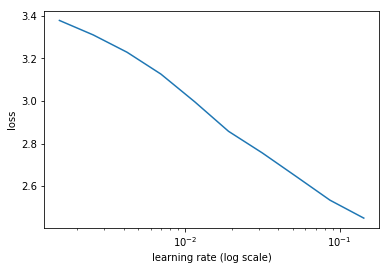#### to change the truncation of the plot use the command below

``````learn.sched.plot(n_skip=5, n_skip_end=1)
``````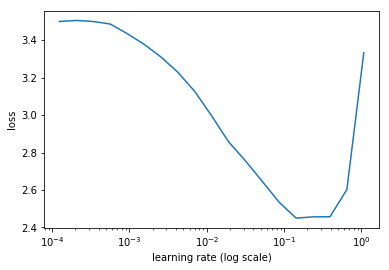#### Set the learning rate

``````lr = 2e-2
``````
``````learn.fit(lr, 1, cycle_len=1)
``````

Failed to display Jupyter Widget of type `HBox`.

If you're reading this message in the Jupyter Notebook or JupyterLab Notebook, it may mean that the widgets JavaScript is still loading. If this message persists, it likely means that the widgets JavaScript library is either not installed or not enabled. See the Jupyter Widgets Documentation for setup instructions.

If you're reading this message in another frontend (for example, a static rendering on GitHub or NBViewer), it may mean that your frontend doesn't currently support widgets.

``````epoch      trn_loss   val_loss   accuracy
0      1.280753   0.604127   0.806941

[0.60412693, 0.8069411069154739]
``````
``````lrs = np.array([lr/1000,lr/100,lr])
``````

### We freeze all layers except the last two layers, find new learning rate and retrain

``````learn.freeze_to(-2)
``````
``````lrf=learn.lr_find(lrs/1000)
learn.sched.plot(1)
``````

Failed to display Jupyter Widget of type `HBox`.

If you're reading this message in the Jupyter Notebook or JupyterLab Notebook, it may mean that the widgets JavaScript is still loading. If this message persists, it likely means that the widgets JavaScript library is either not installed or not enabled. See the Jupyter Widgets Documentation for setup instructions.

If you're reading this message in another frontend (for example, a static rendering on GitHub or NBViewer), it may mean that your frontend doesn't currently support widgets.

`````` 84%|████████▍ | 27/32 [00:08<00:01,  3.26it/s, loss=3.45]
``````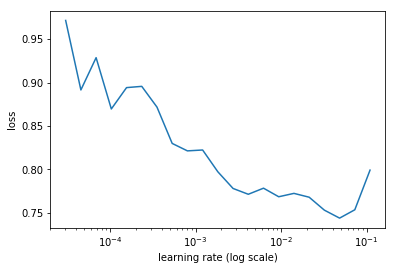``````learn.fit(lrs/5, 1, cycle_len=1)
``````

Failed to display Jupyter Widget of type `HBox`.

If you're reading this message in the Jupyter Notebook or JupyterLab Notebook, it may mean that the widgets JavaScript is still loading. If this message persists, it likely means that the widgets JavaScript library is either not installed or not enabled. See the Jupyter Widgets Documentation for setup instructions.

If you're reading this message in another frontend (for example, a static rendering on GitHub or NBViewer), it may mean that your frontend doesn't currently support widgets.

``````epoch      trn_loss   val_loss   accuracy
0      0.777971   0.556964   0.832782

[0.5569643, 0.8327824547886848]
``````

#### Accuracy is still at 83%

``````learn.unfreeze()
``````

Accuracy isn’t improving much - since many images have multiple different objects, it’s going to be impossible to be that accurate.

``````learn.fit(lrs/5, 1, cycle_len=2)
``````

Failed to display Jupyter Widget of type `HBox`.

If you're reading this message in the Jupyter Notebook or JupyterLab Notebook, it may mean that the widgets JavaScript is still loading. If this message persists, it likely means that the widgets JavaScript library is either not installed or not enabled. See the Jupyter Widgets Documentation for setup instructions.

If you're reading this message in another frontend (for example, a static rendering on GitHub or NBViewer), it may mean that your frontend doesn't currently support widgets.

``````epoch      trn_loss   val_loss   accuracy
0      0.676254   0.546998   0.834285
1      0.460609   0.533741   0.833233

[0.53374064, 0.8332331702113152]
``````
``````learn.save('clas_one')
``````
``````learn.load('clas_one')
``````
``````x,y = next(iter(md.val_dl))
probs = F.softmax(predict_batch(learn.model, x), -1)
x,preds = to_np(x),to_np(probs)
preds = np.argmax(preds, -1)
``````

### Let’s look at the 20 classes

``````fig, axes = plt.subplots(3, 4, figsize=(12, 8))
for i,ax in enumerate(axes.flat):
ima=md.val_ds.denorm(x)[i]
b = md.classes[preds[i]]
ax = show_img(ima, ax=ax)
draw_text(ax, (0,0), b)
plt.tight_layout()
``````
``````Clipping input data to the valid range for imshow with RGB data ([0..1] for floats or [0..255] for integers).
``````

### Note on debuggers

You can use the python debugger `pdb` to step through code.

• `pdb.set_trace()` to set a breakpoint
• `%debug` magic to trace an error

Commands you need to know:

• s / n / c
• u / d
• p
• l
``````> <ipython-input-99-6d7dd6a3d3cc>(4)<module>()
-> ima=md.val_ds.denorm(x)[i]
(Pdb) h

Documented commands (type help <topic>):
========================================
EOF    c          d        h         list      q        rv       undisplay
a      cl         debug    help      ll        quit     s        unt
alias  clear      disable  ignore    longlist  r        source   until
args   commands   display  interact  n         restart  step     up
b      condition  down     j         next      return   tbreak   w
break  cont       enable   jump      p         retval   u        whatis
bt     continue   exit     l         pp        run      unalias  where

Miscellaneous help topics:
==========================
exec  pdb
``````

Can view variables throughout the debugging process

``````(Pdb) n # will go to next step

(Pdb) l # will show the currentlocation
1  	fig, axes = plt.subplots(3, 4, figsize=(12, 8))
2  	for i,ax in enumerate(axes.flat):
3  	    pdb.set_trace()
4  ->	    ima=md.val_ds.denorm(x)[i]
5  	    b = md.classes[preds[i]]
6  	    ax = show_img(ima, ax=ax)
7  	    draw_text(ax, (0,0), b)
8  	plt.tight_layout()
[EOF]

(Pdb) s # will go into a function

(Pdb) c # continue to next break point
``````

# Next Stage : Create a bounding box around an object

We know we can make a regression nn instead of a classification. This is accomplished by changing the last layer of the NN. Instead of Softmax, and use MSE, it is now a regression problem. We can have multiple outputs.

So what we will do is a multiple regression to predict the following values:

• top left x
• top left y
• lower right x
• lower right y

#### But what about the loss function?

``````BB_CSV = PATH/'tmp/bb.csv'
``````

#### Transform the bounding box data

``````bb = np.array([trn_lrg_anno[o] for o in trn_ids])
bbs = [' '.join(str(p) for p in o) for o in bb]

df = pd.DataFrame({'fn': [trn_fns[o] for o in trn_ids], 'bbox': bbs}, columns=['fn','bbox'])
df.to_csv(BB_CSV, index=False)
``````
``````BB_CSV.open().readlines()[:5]
``````
``````['fn,bbox\n',
'000012.jpg,96 155 269 350\n',
'000017.jpg,77 89 335 402\n',
'000023.jpg,1 2 461 242\n',
'000026.jpg,124 89 211 336\n']
``````

### Set our model and parameters

``````f_model=resnet34
sz=224
bs=64
``````

### Tell `fast.ai` to make a continous network model

Set `continuous=True` to tell fastai this is a regression problem, which means it won’t one-hot encode the labels, and will use MSE as the default crit.

Note that we have to tell the transforms constructor that our labels are coordinates, so that it can handle the transforms correctly.

Also, we use CropType.NO because we want to ‘squish’ the rectangular images into squares, rather than center cropping, so that we don’t accidentally crop out some of the objects. (This is less of an issue in something like imagenet, where there is a single object to classify, and it’s generally large and centrally located).

``````tfms = tfms_from_model(f_model, sz, crop_type=CropType.NO, tfm_y=TfmType.COORD)
md = ImageClassifierData.from_csv(PATH, JPEGS, BB_CSV, tfms=tfms, continuous=True)
``````
``````x,y=next(iter(md.val_dl))
``````
``````ima=md.val_ds.denorm(to_np(x))
b = bb_hw(to_np(y)); b
``````
``````array([ 49.,   0., 131., 205.], dtype=float32)
``````

#### Let’s look at an example image with box

``````ax = show_img(ima)
draw_rect(ax, b)
draw_text(ax, b[:2], 'label')
``````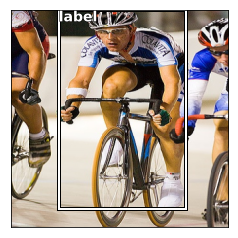### How add additional layers on the end of the Resnet (`custom head`)

fastai let’s you use a `custom_head` to add your own module on top of a convnet, instead of the adaptive pooling and fully connected net which is added by default. In this case, we don’t want to do any pooling, since we need to know the activations of each grid cell.

The final layer has 4 activations, one per bounding box coordinate. Our target is continuous, not categorical, so the MSE loss function used does not do any sigmoid or softmax to the module outputs.

``````head_reg4 = nn.Sequential(Flatten(), nn.Linear(25088,4))
learn.crit = nn.L1Loss()
``````

#### Check the Model to see that the additional layer has been addedm

``````# learn.summary()
``````
``````OrderedDict([('Conv2d-1',
OrderedDict([('input_shape', [-1, 3, 224, 224]),
('output_shape', [-1, 64, 112, 112]),
('trainable', False),
('nb_params', 9408)])),
....
....
('ReLU-121',
OrderedDict([('input_shape', [-1, 512, 7, 7]),
('output_shape', [-1, 512, 7, 7]),
('nb_params', 0)])),
('BasicBlock-122',
OrderedDict([('input_shape', [-1, 512, 7, 7]),
('output_shape', [-1, 512, 7, 7]),
('nb_params', 0)])),
('Flatten-123',
OrderedDict([('input_shape', [-1, 512, 7, 7]),
('output_shape', [-1, 25088]),
('nb_params', 0)])),

------------------- New layer ------------------------
('Linear-124',
OrderedDict([('input_shape', [-1, 25088]),
('output_shape', [-1, 4]),
('trainable', True),
('nb_params', 100356)]))])
------------------- New layer ------------------------
``````

### Try and fit the model

``````learn.lr_find(1e-5,100)
learn.sched.plot(5)
``````

Failed to display Jupyter Widget of type `HBox`.

If you're reading this message in the Jupyter Notebook or JupyterLab Notebook, it may mean that the widgets JavaScript is still loading. If this message persists, it likely means that the widgets JavaScript library is either not installed or not enabled. See the Jupyter Widgets Documentation for setup instructions.

If you're reading this message in another frontend (for example, a static rendering on GitHub or NBViewer), it may mean that your frontend doesn't currently support widgets.

`````` 78%|███████▊  | 25/32 [00:04<00:01,  5.36it/s, loss=475]
``````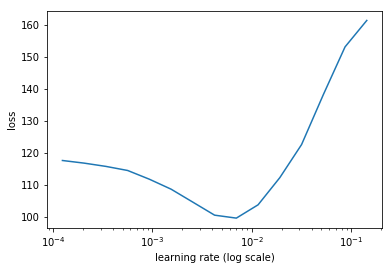#### Set the learning rate

``````lr = 2e-3
``````

#### Train

``````learn.fit(lr, 2, cycle_len=1, cycle_mult=2)
``````

Failed to display Jupyter Widget of type `HBox`.

If you're reading this message in the Jupyter Notebook or JupyterLab Notebook, it may mean that the widgets JavaScript is still loading. If this message persists, it likely means that the widgets JavaScript library is either not installed or not enabled. See the Jupyter Widgets Documentation for setup instructions.

If you're reading this message in another frontend (for example, a static rendering on GitHub or NBViewer), it may mean that your frontend doesn't currently support widgets.

``````epoch      trn_loss   val_loss
0      50.135777  34.477402
1      37.689602  29.124092
2      31.387475  27.658106

[27.658106]
``````
``````lrs = np.array([lr/100,lr/10,lr])
``````
``````learn.freeze_to(-2)
``````
``````lrf=learn.lr_find(lrs/1000)
learn.sched.plot(1)
``````

Failed to display Jupyter Widget of type `HBox`.

If you're reading this message in the Jupyter Notebook or JupyterLab Notebook, it may mean that the widgets JavaScript is still loading. If this message persists, it likely means that the widgets JavaScript library is either not installed or not enabled. See the Jupyter Widgets Documentation for setup instructions.

If you're reading this message in another frontend (for example, a static rendering on GitHub or NBViewer), it may mean that your frontend doesn't currently support widgets.

``````epoch      trn_loss   val_loss
0      80.37384   175370041032704.0
``````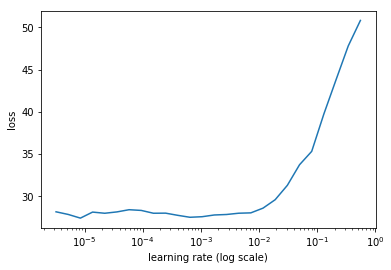``````learn.fit(lrs, 2, cycle_len=1, cycle_mult=2)
``````

Failed to display Jupyter Widget of type `HBox`.

If you're reading this message in the Jupyter Notebook or JupyterLab Notebook, it may mean that the widgets JavaScript is still loading. If this message persists, it likely means that the widgets JavaScript library is either not installed or not enabled. See the Jupyter Widgets Documentation for setup instructions.

If you're reading this message in another frontend (for example, a static rendering on GitHub or NBViewer), it may mean that your frontend doesn't currently support widgets.

``````epoch      trn_loss   val_loss
0      25.814654  23.127014
1      21.655237  21.125538
2      17.600573  20.209145

[20.209145]
``````
``````learn.freeze_to(-3)
``````
``````learn.fit(lrs, 1, cycle_len=2)
``````

Failed to display Jupyter Widget of type `HBox`.

If you're reading this message in the Jupyter Notebook or JupyterLab Notebook, it may mean that the widgets JavaScript is still loading. If this message persists, it likely means that the widgets JavaScript library is either not installed or not enabled. See the Jupyter Widgets Documentation for setup instructions.

If you're reading this message in another frontend (for example, a static rendering on GitHub or NBViewer), it may mean that your frontend doesn't currently support widgets.

``````epoch      trn_loss   val_loss
0      16.644847  21.78323
1      14.667386  20.380457

[20.380457]
``````

#### Save our Model

``````learn.save('reg4')
``````
``````learn.load('reg4')
``````

## Let’s see how our model did!

Anytime there’s a single subject, our model does decent. When there’s multiple objects our model doesn’t perform as well. Next week we will improve our model

``````x,y = next(iter(md.val_dl))
learn.model.eval()
preds = to_np(learn.model(VV(x)))
``````
``````fig, axes = plt.subplots(3, 4, figsize=(12, 8))
for i,ax in enumerate(axes.flat):
ima=md.val_ds.denorm(to_np(x))[i]
b = bb_hw(preds[i])
ax = show_img(ima, ax=ax)
draw_rect(ax, b)
plt.tight_layout()
``````
``````Clipping input data to the valid range for imshow with RGB data ([0..1] for floats or [0..255] for integers).
``````

61 Likes

Part 2 Lesson 8 wiki
Deep Learning Brasília - Lição 8
Deep Learning Brasília - Lição 8
#2

Wow, Thank you for summerizing that! May I suggest to add references to video timestamps, if only that’s not too much work? Regardlessly, this work is superb!

0 Likes

(Tony Holdroyd) #4

Thanks for doing that, it must have taken a while, very useful.

0 Likes

(Sanyam Bhutani) #5

Our saviour @timlee is back.2 Likes

2 Likes

Many thanks for the great notes @timlee. One correction: `open_image` is from fastai, not PIL. Underneath it uses opencv (`cv2`) . It’s the function we looked at the implementation of in vscode.

A clarification: pathlib isn’t just for creating a generator - that’s what the `iterdir` method does. pathlib does a lot more (e.g the `/` operator, the `open` method we used to open the json file, etc).

3 Likes

(Amrit ) #8

@timlee really glad to see your notes again. Thanks!0 Likes

(Ankit Goila) #9

This is great! As always, thanks for putting these together @timlee0 Likes

(Mandar Deshpande) #10

After working through the week’s notebook ones after going through my notes, and then a second pass along with your notes always helps in bolstering the conceptual understanding.

@timlee really a big thanks to you from us all for compiling and explaining the lessons again in such detail!0 Likes

(Avinash) #11

Thanks for the notes @timlee. As usual, it’s detailed and useful.

0 Likes

(Tim Lee) #12

Thanks for the feedback. I’ve updated the text according!

0 Likes

(Bart Fish) #13

It’s all greek to me.

Posted this here because I’m not sure where the wiki is for this lesson. But anyway ,here’s a pretty good link for both Greek and Mathematical notation.
https://www.rapidtables.com/math/symbols/Basic_Math_Symbols.html

0 Likes

You can edit the ‘in class discussion’ post.

0 Likes

(Bart Fish) #15

did that, should I withdraw the post?

0 Likes

No need - it’s nice to have both I think.

0 Likes

(Evgeny Sushko) #17

Thank you for putting it together @timlee! That’s very helpful.

0 Likes

(KRO) #18

Does anyone know where predict_batch is defined?

It’s used in these notes where the model is tested under “Largest item classifier” in

probs = F.softmax(predict_batch(learn.model, x), -1)

I tried jupyter notebook’s shift-tab and ?predict_batch, as well as googling and also just trying grep -R for it in the fastai dir in bash, but no luck.

1 Like

Its a function in fastai.model. Try out using VS Code as Jeremy was showing in the lecture, very useful for digging around in the source code.

0 Likes

(Suvash Thapaliya) #20

You probably haven’t updated(git pull) fastai repo.
Also, easily searchable on Github. https://github.com/fastai/fastai/search?utf8=✓&q=predict_batch&type=

3 Likes

Part 2 Lesson 8 wiki
(Sharwon Pius) #21

You can try this out !

1 Like